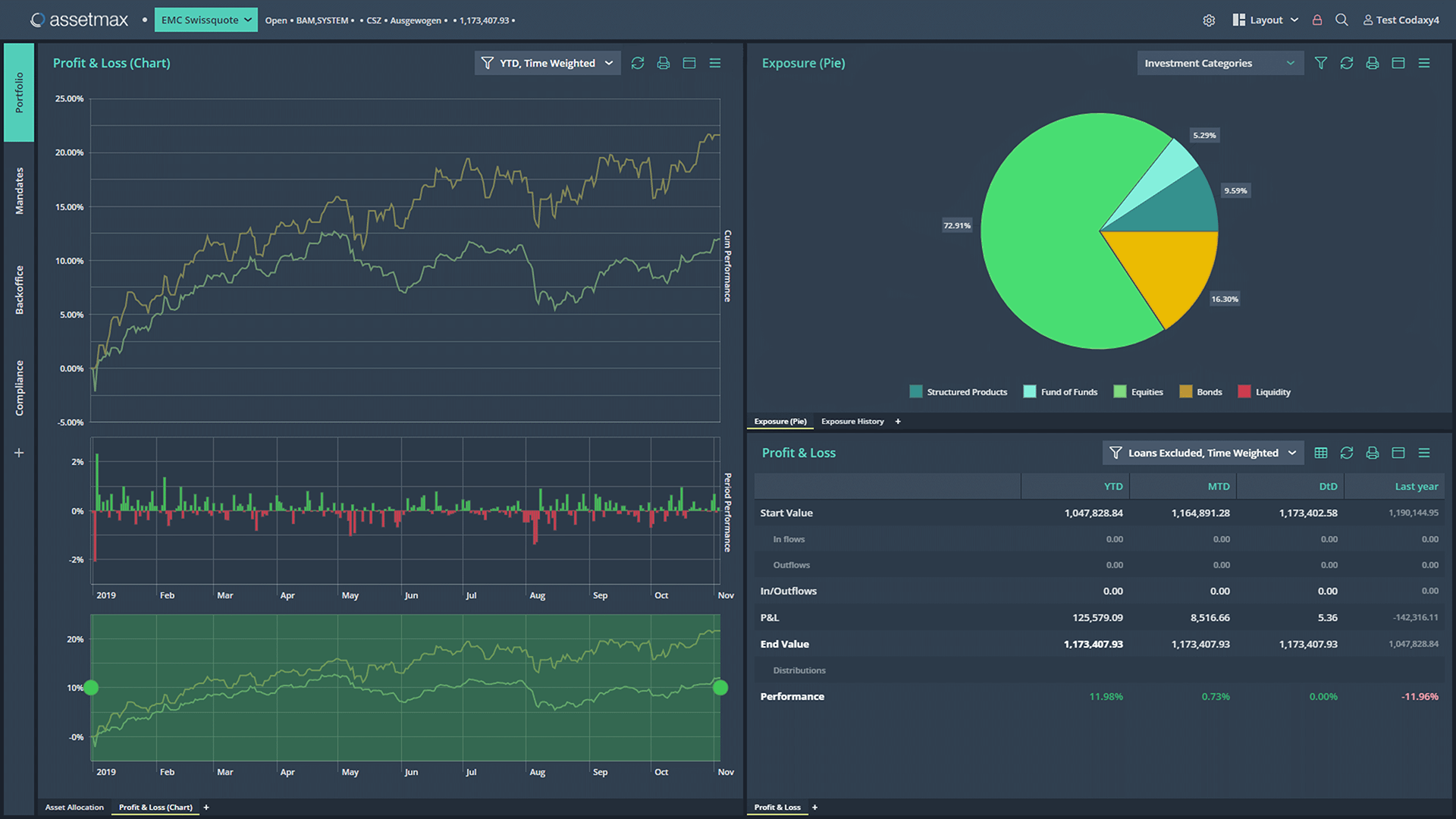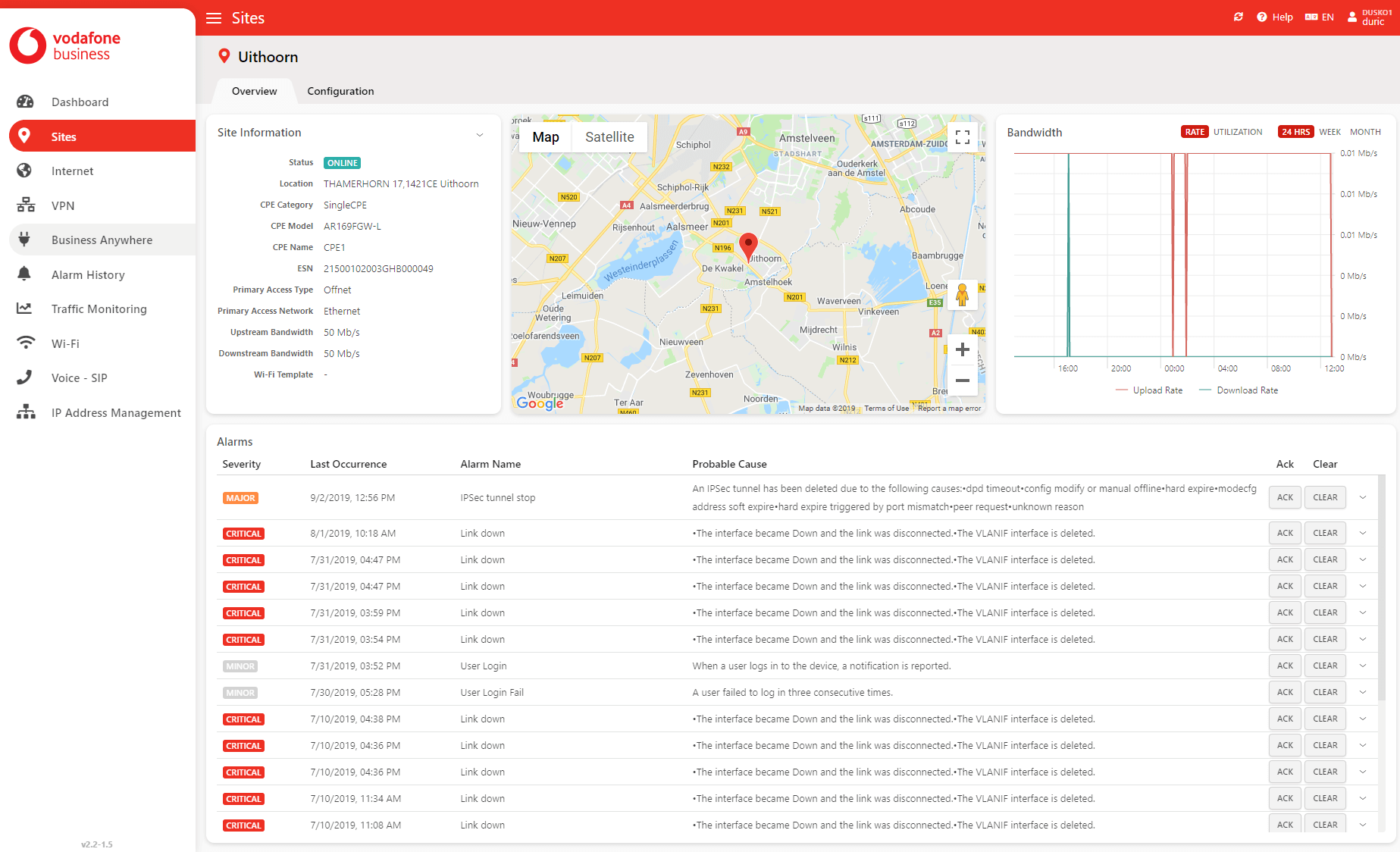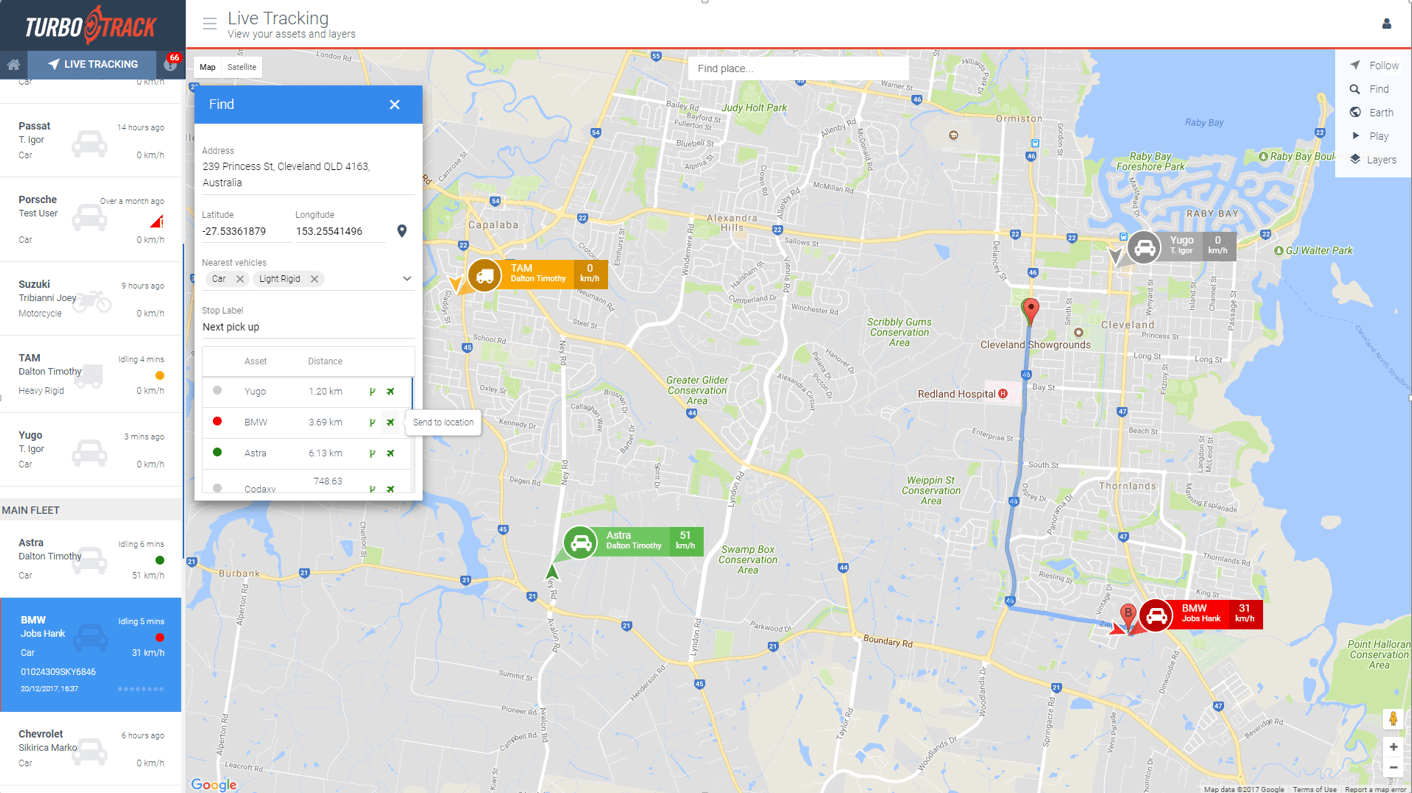We believe that
We believe that
telco  network management  e-commerce  smart home  financial  administrative  stock trading  legal  wealth management  crypto  fleet tracking
telco  network management  e-commerce  smart home  financial  administrative  stock trading  legal  wealth management  crypto  fleet tracking
```import { Controller } from "cx/ui";

const day = 24 * 60 * 60 * 1000;
let startDate = Date.now() - 30 * day;
let categories = {
Opened: 12,
Fixed: 7,
Verified: 10
};

let series = [];

for (let cat in categories) {
let points = [];

let v = Math.round(15 * Math.random());

for (let i = 0; i < 30; i++) {
v += Math.round(4 * (Math.random() - 0.5));
if (v < 0) v = 0;
points.push({
date: startDate + i * day,
count: 15 + Math.round(20 * Math.random())
});
}

series.push({
category: cat,
data: points,
color: categories[cat],
trackedValue: null
});
}

export default class extends Controller {
onInit() {
this.store.init("series", series);
}
} ```
```import { Controller } from "cx/ui";

const day = 24 * 60 * 60 * 1000;
let startDate = Date.now() - 30 * day;
let categories = {
Opened: 12,
Fixed: 7,
Verified: 10
};

let series = [];

for (let cat in categories) {
let points = [];

let v = Math.round(15 * Math.random());

for (let i = 0; i < 30; i++) {
v += Math.round(4 * (Math.random() - 0.5));
if (v < 0) v = 0;
points.push({
date: startDate + i * day,
count: 15 + Math.round(20 * Math.random())
});
}

series.push({
category: cat,
data: points,
color: categories[cat],
trackedValue: null
});
}

export default class extends Controller {
onInit() {
this.store.init("series", series);
}
} ```
applications should be
```import { Controller } from "cx/ui";

const day = 24 * 60 * 60 * 1000;
let startDate = Date.now() - 30 * day;
let categories = {
Opened: 12,
Fixed: 7,
Verified: 10
};

let series = [];

for (let cat in categories) {
let points = [];

let v = Math.round(15 * Math.random());

for (let i = 0; i < 30; i++) {
v += Math.round(4 * (Math.random() - 0.5));
if (v < 0) v = 0;
points.push({
date: startDate + i * day,
count: 15 + Math.round(20 * Math.random())
});
}

series.push({
category: cat,
data: points,
color: categories[cat],
trackedValue: null
});
}

export default class extends Controller {
onInit() {
this.store.init("series", series);
}
} ```
```import { Controller } from "cx/ui";

const day = 24 * 60 * 60 * 1000;
let startDate = Date.now() - 30 * day;
let categories = {
Opened: 12,
Fixed: 7,
Verified: 10
};

let series = [];

for (let cat in categories) {
let points = [];

let v = Math.round(15 * Math.random());

for (let i = 0; i < 30; i++) {
v += Math.round(4 * (Math.random() - 0.5));
if (v < 0) v = 0;
points.push({
date: startDate + i * day,
count: 15 + Math.round(20 * Math.random())
});
}

series.push({
category: cat,
data: points,
color: categories[cat],
trackedValue: null
});
}

export default class extends Controller {
onInit() {
this.store.init("series", series);
}
} ```
well-coded
beautiful
easy to use
```import { Controller } from "cx/ui";

const day = 24 * 60 * 60 * 1000;
let startDate = Date.now() - 30 * day;
let categories = {
Opened: 12,
Fixed: 7,
Verified: 10
};

let series = [];

for (let cat in categories) {
let points = [];

let v = Math.round(15 * Math.random());

for (let i = 0; i < 30; i++) {
v += Math.round(4 * (Math.random() - 0.5));
if (v < 0) v = 0;
points.push({
date: startDate + i * day,
count: 15 + Math.round(20 * Math.random())
});
}

series.push({
category: cat,
data: points,
color: categories[cat],
trackedValue: null
});
}

export default class extends Controller {
onInit() {
this.store.init("series", series);
}
} ```
We believe that We believe that  telco  telco
applications should be  applications should be  well-coded  well-coded
telco
network management
e-commerce
smart home
financial
legal
wealth management
crypto
fleet tracking
Web and Mobile Applications

# We develop

well-coded, beautiful and easy to use

# The new face of Assetmax

We helped Assetmax AG to upgrade their application's user interface while preserving all functionalityUI & UX DesignFront-End Development
```import { Controller } from "cx/ui";

const day = 24 * 60 * 60 * 1000;
let startDate = Date.now() - 30 * day;
let categories = {
Opened: 12,
Fixed: 7,
Verified: 10
};

let series = [];

for (let cat in categories) {
let points = [];

let v = Math.round(15 * Math.random());

for (let i = 0; i < 30; i++) {
v += Math.round(4 * (Math.random() - 0.5));
if (v < 0) v = 0;
points.push({
date: startDate + i * day,
count: 15 + Math.round(20 * Math.random())
});
}

series.push({
category: cat,
data: points,
color: categories[cat],
trackedValue: null
});
}

export default class extends Controller {
onInit() {
this.store.init("series", series);
}
} ```# Empower businessusers to easilymanage their ownnetwork

Vodafone Flex makes it possible to easily activate, change and deactivate network services across the world based on SD-WAN technologyUI & UX DesignFront-End Development
```import { Controller } from "cx/ui";

const day = 24 * 60 * 60 * 1000;
let startDate = Date.now() - 30 * day;
let categories = {
Opened: 12,
Fixed: 7,
Verified: 10
};

let series = [];

for (let cat in categories) {
let points = [];

let v = Math.round(15 * Math.random());

for (let i = 0; i < 30; i++) {
v += Math.round(4 * (Math.random() - 0.5));
if (v < 0) v = 0;
points.push({
date: startDate + i * day,
count: 15 + Math.round(20 * Math.random())
});
}

series.push({
category: cat,
data: points,
color: categories[cat],
trackedValue: null
});
}

export default class extends Controller {
onInit() {
this.store.init("series", series);
}
} ```# A new way into Bitcoin

We joined forces with Rise Inc to create the most intuitive Bitcoin wallet on the marketFront-End DevelopmentBack-End Development
```import { Controller } from "cx/ui";

const day = 24 * 60 * 60 * 1000;
let startDate = Date.now() - 30 * day;
let categories = {
Opened: 12,
Fixed: 7,
Verified: 10
};

let series = [];

for (let cat in categories) {
let points = [];

let v = Math.round(15 * Math.random());

for (let i = 0; i < 30; i++) {
v += Math.round(4 * (Math.random() - 0.5));
if (v < 0) v = 0;
points.push({
date: startDate + i * day,
count: 15 + Math.round(20 * Math.random())
});
}

series.push({
category: cat,
data: points,
color: categories[cat],
trackedValue: null
});
}

export default class extends Controller {
onInit() {
this.store.init("series", series);
}
} ``````import { Controller } from "cx/ui";

const day = 24 * 60 * 60 * 1000;
let startDate = Date.now() - 30 * day;
let categories = {
Opened: 12,
Fixed: 7,
Verified: 10
};

let series = [];

for (let cat in categories) {
let points = [];

let v = Math.round(15 * Math.random());

for (let i = 0; i < 30; i++) {
v += Math.round(4 * (Math.random() - 0.5));
if (v < 0) v = 0;
points.push({
date: startDate + i * day,
count: 15 + Math.round(20 * Math.random())
});
}

series.push({
category: cat,
data: points,
color: categories[cat],
trackedValue: null
});
}

export default class extends Controller {
onInit() {
this.store.init("series", series);
}
} ``````import { Controller } from "cx/ui";

const day = 24 * 60 * 60 * 1000;
let startDate = Date.now() - 30 * day;
let categories = {
Opened: 12,
Fixed: 7,
Verified: 10
};

let series = [];

for (let cat in categories) {
let points = [];

let v = Math.round(15 * Math.random());

for (let i = 0; i < 30; i++) {
v += Math.round(4 * (Math.random() - 0.5));
if (v < 0) v = 0;
points.push({
date: startDate + i * day,
count: 15 + Math.round(20 * Math.random())
});
}

series.push({
category: cat,
data: points,
color: categories[cat],
trackedValue: null
});
}

export default class extends Controller {
onInit() {
this.store.init("series", series);
}
} ```# Keep an eye on your cars and trucks

Comm8 needed help with a complete rewrite of their proprietary vehicle tracking software Turbo TrackUI & UX DesignFront-End DevelopmentBack-End Development
```import { Controller } from "cx/ui";

const day = 24 * 60 * 60 * 1000;
let startDate = Date.now() - 30 * day;
let categories = {
Opened: 12,
Fixed: 7,
Verified: 10
};

let series = [];

for (let cat in categories) {
let points = [];

let v = Math.round(15 * Math.random());

for (let i = 0; i < 30; i++) {
v += Math.round(4 * (Math.random() - 0.5));
if (v < 0) v = 0;
points.push({
date: startDate + i * day,
count: 15 + Math.round(20 * Math.random())
});
}

series.push({
category: cat,
data: points,
color: categories[cat],
trackedValue: null
});
}

export default class extends Controller {
onInit() {
this.store.init("series", series);
}
} ```# What we can do for you

We’re highly specialized in web-oriented business applications such as online trading software, cloud management consoles, Saas applications, IoT dashboards, accounting and back-office systems, etc.### Front-end Development

JavaScript is our home territory. We make beautiful new interfaces in a blink of an eye. Does your application need a facelift?### Back-end Development

Using .NET and Node.js we build scalable and maintainable applications that withstand the test of time.### UI & UX Design

We deeply care about design. Our design team transforms ideas into beautiful and easy to use products.

# What’s our secret?

We are keeping track of the latest technology trends and integrating best bits and pieces into CxJS - a comprehensive UI framework built for enterprise applications.

Visit cxjs.io